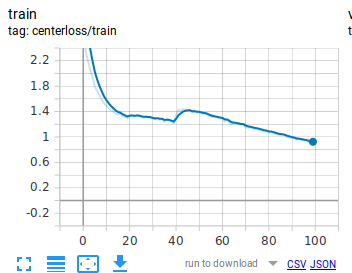# Decaying learning rate spikes center loss

Hello,

I am implementing centerloss in my application. Center loss is introduced in ECCV2016: A Discriminative Feature Learning Approach for Deep Face Recognition. The idea is to cluster features (embeddings) before the last FC layer. This means embeddings’ distances to their cluster center will be reduced using centerloss. centerloss is optimized jointly with crossentropy. So as crossentropy tries to separate features, centerloss will make features of the same class close to each other. At each epoch centers are updated based on the average of embeddings. Centerloss has been implemented by many. I’m mainly using the implementation here with minor tweaks: PyTorch CenterLoss. Here is the implementation:

``````class CenterLoss(nn.Module):
def __init__(self, num_classes, feat_dim, size_average=True):
super(CenterLoss, self).__init__()
self.centers = nn.Parameter(torch.FloatTensor(num_classes, feat_dim))
self.centerloss = CenterLossFunction.apply
self.feat_dim = feat_dim
self.size_average = size_average
self.reset_params()

def reset_params(self):
nn.init.kaiming_normal_(self.centers.data.t())

def forward(self, feat, label):
batch_size = feat.size(0)
feat = feat.view(batch_size, -1)
# To check the dim of centers and features
if feat.size(1) != self.feat_dim:
raise ValueError("Center's dim: {0} should be equal to input feature's \
dim: {1}".format(self.feat_dim,feat.size(1)))
batch_size_tensor = feat.new_empty(1).fill_(batch_size if self.size_average else 1)
loss = self.centerloss(feat, label, self.centers, batch_size_tensor)
return loss

class CenterLossFunction(Function):
@staticmethod
def forward(ctx, feature, label, centers, batch_size):
ctx.save_for_backward(feature, label, centers, batch_size)
centers_batch = centers.index_select(0, label.long())
return (feature - centers_batch).pow(2).sum() / 2.0 / batch_size

@staticmethod
feature, label, centers, batch_size = ctx.saved_tensors
centers_batch = centers.index_select(0, label.long())
diff = centers_batch - feature
# init every iteration
counts = centers.new_ones(centers.size(0))
ones = centers.new_ones(label.size(0))

``````

In my application (image classification), I have training, validation and test sets. Here is my main script using centerloss optimized jointly with crossentropy:

``````def main(cfg):

# ---------------

# Create Model
# ------------
model = resnet18(in_channels=1, num_classes=8)
model = torch.nn.DataParallel(model).to(device)

# define loss function (criterion) and optimizer
# ----------------------------------------------
criterion = {}
optimizer = {}
criterion['xent'] = nn.CrossEntropyLoss().to(device)
optimizer['xent'] = torch.optim.SGD(model.parameters(), cfg['lr'],
momentum=cfg['momentum'],
weight_decay=cfg['weight_decay'])

criterion['center'] = CenterLoss(8, 512).to(device)
optimizer['center'] = torch.optim.SGD(criterion['center'].parameters(), cfg['alpha'])

# training and evaluation
# -----------------------
for epoch in range(cfg['epochs']):
# train for one epoch
train(train_loader, model, criterion, optimizer, epoch, cfg)
# evaluate on validation set
# evaluate on test set

def train(train_loader, model, criterion, optimizer, epoch, cfg):
losses = {}
losses['xent'] = AverageMeter()
losses['center'] = AverageMeter()
losses['loss'] = AverageMeter()
train_acc = AverageMeter()
y_pred, y_true, y_scores = [], [], []

# switch to train mode
model.train()

end = time.time()
for i, (images, target) in enumerate(train_loader):

images = images.to(device)
target = target.to(device)
# compute output
feat, output = model(images)
xent_loss = criterion['xent'](output, target)
center_loss = criterion['center'](feat, target)
loss = xent_loss + cfg['lamb'] * center_loss

# measure accuracy and record loss
acc, pred = accuracy(output, target)
losses['xent'].update(xent_loss.item(), images.size(0))
losses['center'].update(center_loss.item(), images.size(0))
losses['loss'].update(loss.item(), images.size(0))
train_acc.update(acc.item(), images.size(0))

# collect for other measures
y_pred.append(pred)
y_true.append(target)
y_scores.append(output.data)

# compute gradient and do SGD step
loss.backward()
optimizer['xent'].step()
optimizer['center'].step()

m = metrics(y_pred, y_true, y_scores)
progress = (...)
tb_write(losses, train_acc.avg, m, epoch, writer=writer, tag='train')

def validate(valid_loader, model, criterion, epoch, cfg):
losses = {}
losses['xent'] = AverageMeter()
losses['center'] = AverageMeter()
losses['loss'] = AverageMeter()
valid_acc = AverageMeter()
y_pred, y_true, y_scores = [], [], []

# switch to evaluate mode
model.eval()

for i, (images, target) in enumerate(valid_loader):

images = images.to(device)
target = target.to(device)

# compute output
feat, output = model(images)
xent_loss = criterion['xent'](output, target)
center_loss = criterion['center'](feat, target)
loss = xent_loss + cfg['lamb'] * center_loss

# measure accuracy and record loss
acc, pred = accuracy(output, target)
losses['xent'].update(xent_loss.item(), images.size(0))
losses['center'].update(center_loss.item(), images.size(0))
losses['loss'].update(loss.item(), images.size(0))
valid_acc.update(acc.item(), images.size(0))

# collect for other measures
y_pred.append(pred)
y_true.append(target)
y_scores.append(output.data)

m = metrics(y_pred, y_true, y_scores)
progress = (...)
tb_write(losses, valid_acc.avg, m, epoch, writer=writer, tag='valid')

def test(test_loader, model, criterion, epoch, cfg):
'''
the same as validation
'''

if epoch < 20:
lr = cfg['lr']
elif 20 <= epoch < 40:
lr = cfg['lr'] / 2.0
else:
lr = cfg['lr'] / 10.0
for param_group in optimizer.param_groups:
param_group['lr'] = lr

if __name__ == '__main__':
I use `lamb=0.01`, `alpha=0.5` for centerloss and an ‘lr=0.05’ for my crossentropy SGD optimizer. I decay learning rate by 2.0 at epoch 20 and by a factor of 10.0 at epoch 40. When I’m logging my scalars: